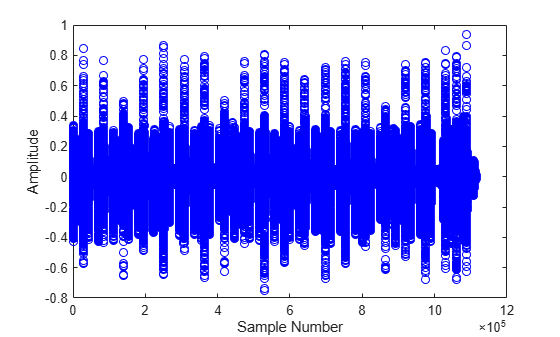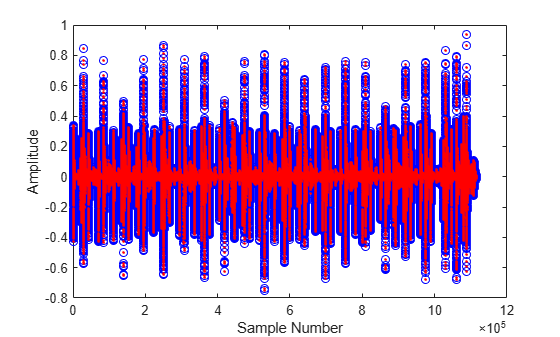Documentation

imdct

Inverse modified discrete cosine transform

Description

example

X = imdct(Y,win) returns the inverse modified discrete cosine transform (IMDCT) of Y, followed by multiplication with time window win and overlap-addition of the frames with 50% overlap.

X = imdct(Y,win,Name,Value) sets each property Name to the specified Value. Unspecified properties have default values.

Examples

collapse all

Read in an audio file, convert it to mono, and then plot it.

audioIn = mean(audioIn,2);

figure(1)
plot(audioIn,'bo')
ylabel('Amplitude')
xlabel('Sample Number')Calculate the MDCT using a 4096-point sine window. Plot the power of the MDCT coefficients over time.

N = 4096;
wdw = sin(pi*((1:N)-0.5)/N);

C = mdct(audioIn,wdw);

figure(2)
surf(20*log10(C.*conj(C)),'EdgeColor','none');
view([0 90])
xlabel('Frame')
ylabel('Frequency')
axis([0 size(C,2) 0 size(C,1)])
colorbarTransform the representation back to the time domain. Verify the perfect reconstruction property by computing the mean squared error. Plot the reconstructed signal over the original signal.

audioReconstructed = imdct(C,wdw);
err = mean((audioIn-audioReconstructed(1:size(audioIn,1),:)).^2)
err = 9.5933e-31
figure(1)
hold on
plot(audioReconstructed,'r.')
ylabel('Amplitude')
xlabel('Sample Number')To enable perfect reconstruction, the mdct function zero-pads the front and back of the audio input signal. The signal returned from imdct removes the zero padding added for perfect reconstruction.

Read in an audio file, create a 2048-point Kaiser-Bessel-derived window, and then clip the audio signal so that its length is a multiple of 2048.

win = kbdwin(2048);

xClipped = x(1:end - rem(size(x,1),numel(win)));

Convert the signal to the frequency domain, and then reconstruct it back in the time domain. Plot the original and reconstructed signals and display the reconstruction error.

C = mdct(xClipped,win);
y = imdct(C,win);

figure(1)
t = (0:size(xClipped,1)-1)'/fs;
plot(t,xClipped,'bo',t,y,'r.')
legend('Original Signal','Reconstructed Signal')
title(strcat("Reconstruction Error = ",num2str(mean((xClipped-y).^2))))
xlabel('Time (s)')
ylabel('Amplitude')You can perform the MDCT and IMDCT without input padding using the PadInput name-value pair. However, there will be a reconstruction error in the first half-frame and last half-frame of the signal.

figure(2)
t = (0:size(xClipped,1)-1)'/fs;
plot(t,xClipped,'bo',t,y,'r.')
legend('Original Signal','Reconstructed Signal')
title(strcat("Reconstruction Error (Without Input Padding) = ",num2str(mean((xClipped-y).^2))))
xlabel('Time (s)')
ylabel('Amplitude')If you specify an input signal to the mdct that is not a multiple of the window length, then the input signal is padded with zeros. Pass the original unclipped signal through the transform pair and compare the original signal and the reconstructed signal.

C = mdct(x,win);
y = imdct(C,win);

figure(3)

subplot(2,1,1)
plot(x)
title('Original Signal')
ylabel('Amplitude')
axis([0,max(size(y,1),size(x,1)),-0.5,0.5])

subplot(2,1,2)
plot(y)
title('Reconstructed Signal')
xlabel('Time (s)')
ylabel('Amplitude')
axis([0,max(size(y,1),size(x,1)),-0.5,0.5])The reconstructed signal is padded with zeros at the back end. Remove the zero-padding from the reconstructed signal, plot the original and reconstructed signal, and then display the reconstruction error.

figure(4)
y = y(1:size(x,1));
t = (0:size(x,1)-1)'/fs;
plot(t,x,'bo',t,y,'r.')
legend('Original Signal','Reconstructed Signal')
title(strcat("Reconstruction Error = ",num2str(mean((x-y).^2))))
xlabel('Time (s)')
ylabel('Amplitude')Create a dsp.AudioFileReader object to read in audio data frame-by-frame. Create a dsp.SignalSink to log the reconstructed signal for comparison. Create a dsp.AsyncBuffer to buffer the input stream.

logger = dsp.SignalSink;
buff = dsp.AsyncBuffer;

Create a 512-point Kaiser-Bessel-derived window.

N = 512;
win = kbdwin(N);

In an audio stream loop:

1. Read a frame of data from the file.

2. Write the frame of data to the async buffer.

3. If half a frame of data is present, read from the buffer and then perform the transform pair. Overlap-add the current output from imdct with the previous output, and log the results. Update the memory.

mem = zeros(N/2,2); % initialize an empty memory

write(buff,audioIn);

while buff.NumUnreadSamples >= N/2

logger(y(1:N/2,:)+mem)
mem = y(N/2+1:end,:);
end

end

% Perform the transform pair one last time with a zero-padded final signal.
logger(y(1:N/2,:)+mem)

reconstructedSignal = logger.Buffer;

Read in the entire original audio signal. Trim the front and back zero padding from the reconstructed signal for comparison. Plot one channel of the original and reconstructed signals and display the reconstruction error.

signalLength = size(originalSignal,1);
reconstructedSignal = reconstructedSignal((N/2+1):(N/2+1)+signalLength-1,:);

t = (0:size(originalSignal,1)-1)'/fs;
plot(t,originalSignal(:,1),'bo',t,reconstructedSignal(:,1),'r.')
legend('Original Signal','Reconstructed Signal')
title(strcat("Reconstruction Error = ", ...
num2str(mean((originalSignal-reconstructedSignal).^2,'all'))))
xlabel('Time (s)')
ylabel('Amplitude')Input Arguments

collapse all

Modified discrete cosine transform (MDCT), specified as a vector, matrix, or 3-D array. The dimensions of Y are interpreted as output from the mdct function. If Y is an L-by-M-by-N array, the dimensions are interpreted as:

• L –– Number of points in the frequency-domain representation of each frame. L must be half the number of points in the window, win.

• M –– Number of frames.

• N –– Number of channels.

Data Types: single | double

Window applied in the time domain, specified as vector. The length of win must be twice the number of rows of Y: numel(win)==2*size(Y,1). To enable perfect reconstruction, use the same window used in the forward transformation mdct.

Data Types: single | double

Name-Value Pair Arguments

Specify optional comma-separated pairs of Name,Value arguments. Name is the argument name and Value is the corresponding value. Name must appear inside quotes. You can specify several name and value pair arguments in any order as Name1,Value1,...,NameN,ValueN.

Flag if input to the forward mdct was padded. If set to true, the output is truncated at both ends to remove the zero-padding that the forward mdct added.

Data Types: logical

Output Arguments

collapse all

Inverse modified discrete cosine transform (IMDCT) of input array Y, returned as a column vector or matrix of independent channels.

Data Types: single | double

Algorithms

The inverse modified discrete cosine transform is a time-frequency transform. Given a frequency domain input signal Y and window win, the imdct function performs the follows steps for each independent channel:

1. Each frame of the input is converted into a time-domain representation:

$X\left(n\right)=\sum _{k=0}^{\frac{N}{2}-1}Y\left(k\right)\mathrm{cos}\left[\frac{\pi }{\left(N}{2}\right)}\left(n+\frac{\left(N}{2}\right)+1}{2}\right)\left(k+\frac{1}{2}\right)\right]\text{\hspace{0.17em}},\text{ }n=0,1,...,N-1$

where N is the number of elements in win.

2. Each frame of the time-domain signal is multiplied by the window, win.

3. The frames are overlap-added with 50% overlap to construct a contiguous time-domain signal. If PadInput is set to true, the imdct function assumes the original input signal in the forward transform (mdct) was padded with N/2 zeros on the front and back and removes the padding. By default, PadInput is set to true.

 Princen, J., A. Johnson, and A. Bradley. "Subband/Transform Coding Using Filter Bank Designs Based on Time Domain Aliasing Cancellation." IEEE International Conference on Acoustics, Speech, and Signal Processing (ICASSP). 1987, pp. 2161–2164.

 Princen, J., and A. Bradley. "Analysis/Synthesis Filter Bank Design Based on Time Domain Aliasing Cancellation." IEEE Transactions on Acoustics, Speech, and Signal Processing. Vol. 34, Issue 5, 1986, pp. 1153–1161.

Audio Toolbox DocumentationGet trial now